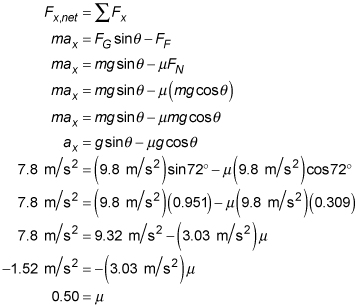##### Physics I: 501 Practice Problems For Dummies (+ Free Online Practice)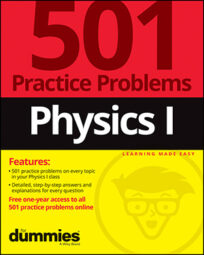Using physics, you can apply Newton's laws of motion to describe how friction affects the way objects move on an incline. Free-body diagrams are also useful in describing the forces that are at work.

Here are some practice questions that you can try.

## Practice questions

1. A 5-kilogram crate is placed on a 42-degree ramp and immediately begins to slide. How far does it slide in 4 seconds if the force of friction is 30 newtons up the ramp?

2. A block with an initial speed of 1 meter per second slides 5.8 meters down a ramp inclined at 72 degrees in 1.1 seconds. What is the coefficient of friction between the block and the ramp, rounded to the nearest hundredth?

The following are the answers to the practice questions:

1. 4.5 m

Draw a free-body diagram to identify the forces acting along the axis of motion (the ramp's surface):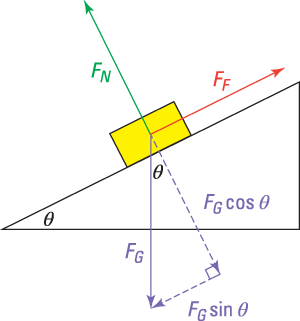Where FN is the normal force, FG is the force of gravity (FG = maG = mg on Earth's surface), and FF is the force of friction. The two forces acting along the ramp's axis are the force of friction and the horizontal (or x) component of the gravitational force. By Newton's second law, the sum of those forces is equal to the crate's mass times its acceleration along the ramp.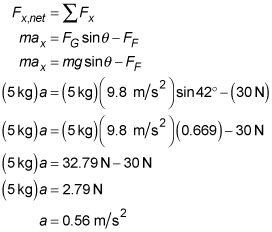Use the displacement formula to calculate how far away the crate is from its starting position after 4 seconds, keeping in mind that the initial velocity is 0 meters per second: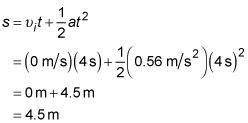2. 0.50

First use the kinematic data to solve for the block's acceleration. Use the displacement formula,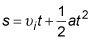where s is the displacement,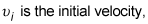a is the acceleration, and t is the amount of elapsed time: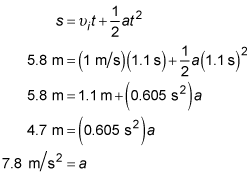As with most every force problem, start by drawing a free-body diagram: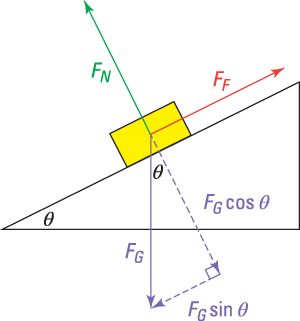Where FN is the normal force, FG is the force of gravity (FG = maG = mg on Earth's surface), and FF is the force of friction. Friction points up because the block slides down, and friction always points in the opposite direction from the motion. Now add up the forces in each direction (x and y) and set their sums equal to the block's mass times its acceleration along that particular axis (ay = 0 meters per second squared because the block doesn't accelerate off the ramp's surface or into it). In the y or vertical direction: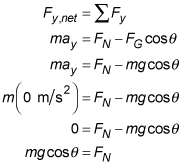And in the x or horizontal direction, where "down the slope" is taken as positive in this solution: